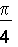﻿ FX Equation

# FX Equation

Top  Previous  Next
 FX Equation is an equation editor that takes the chore of formatting equations away from you.  It is for people who love the output from the modern equation editors but hate using them.  FX Equation automatically formats, with a minimum of input from you, just about all of the equations an average mathematics teacher uses everyday.Video - FX Equation Overview   First a simple example:   If you want a simple equation in your document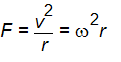normally you would have to load a large and cumbersome equation editor or type the equation into your word processor and manually italicise the variables and superscript the powers.  Alternatively you could load FX Equation and type   F=v2/r=om2r   and press Enter.   FX Equation understands enough about equations to correctly format this for you.   Other examples of equations FX Equation can format include:               Limits                ga=lim(n=>inf)(sigma(k=1,n) 1/k- ln n)=0.5772  gives you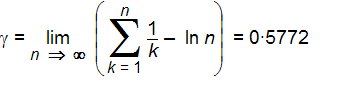Integrals        s(t) = s\0 + int |v(t)| dt   gives you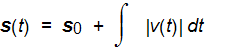Vectors                (3,2) gives you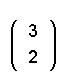Fractions        pi/4 gives you